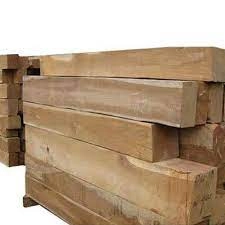Bradley is making a box that has a length of 14 7/8 inches and a width of 5 1/3 inches. How much wood does he need to make the box?

A =  79 13 = 238/3 in2

### Step-by-step explanation:Did you find an error or inaccuracy? Feel free to write us. Thank you!

Tips for related online calculators
Need help calculating sum, simplifying, or multiplying fractions? Try our fraction calculator.
Need help with mixed numbers? Try our mixed-number calculator.

#### Grade of the word problem:

We encourage you to watch this tutorial video on this math problem: